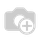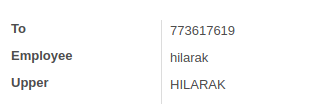# Help

i have 2 field like this

horse_power = fields.Integer()
total_speed = fields.Integer(
string='Speed Total'store=Truecompute='get_total_speed')
@api.depends('horse_power')
def get_total_speed(self):
self.total_speed = self.horse_power * 50

in form view total_speed is readonly but i want to edit that fields.If the field needs to be editable add an inverse function for the computing field. But the intention for an inverse function is not to make it editable. You can rethink your logic and try to achieve with onchange or in write function. Here I am regenerating my previous answer to the same question.

The Use of the Inverse parameter is quite simple. Normally, the computing fields are read-only because it computes the values on the fly from the recordset. If you need to make a manual entry on the computing field, that can be done by giving inverse function. So it triggers call of the decorated function when the field is written/”created”. It reverses the computation and set the relevant fields.

`upper = fields.Char(compute='_compute_upper',                    inverse='_inverse_upper',                    search='_search_upper')@api.depends('employee_id')def _compute_upper(self):    for rec in self:        rec.upper = rec.employee_name.upper() if rec.employee_name else Falsedef _inverse_upper(self):    for rec in self:        rec.employee_name = rec.upper.lower() if rec.upper else False`

1.2. Editing computed field3.  Inverse Function TriggeredUpdate:-

By default, a computed field is not stored in the database and is computed on-the-fly. Adding the attribute ``store=True`` will store the field's values in the database. The advantage of a stored field is that searching on that field is done by the database itself.

So computed fields that are not stored in the database can't be searched normally, To enable searching we have to define the search function explicitly. This can be achieved by adding ''search '' param with the computing field. If we add a method to search on a computed field, the method is invoked when processing domains before doing an actual search on the model.

Another eg :

`standard_price = fields.Float(    'Cost', compute='_compute_standard_price',    inverse='_set_standard_price', search='_search_standard_price',    digits=dp.get_precision('Product Price'), groups="base.group_user",)`
`def _search_standard_price(self, operator, value):    products = self.env['product.product'].search([('standard_price', operator, value)], limit=None)    return [('id', 'in', products.mapped('product_tmpl_id').ids)]`Hi,

For making compute field editable you have to define the inverse function for it. See this: How To Make Compute Field Editable In Odoo

ThanksHello,

whenever you make compute field in odoo it will be automatically readonly. you can not edit it.

if you want to make it editable try following code

horse_power = fields.Integer()
total_speed = fields.Integer(string='Speed Total')
@api.onchange('horse_power')
def get_total_speed(self):
self.total_speed = self.horse_power * 50

Thanks & Regards

Ankit Vaghela